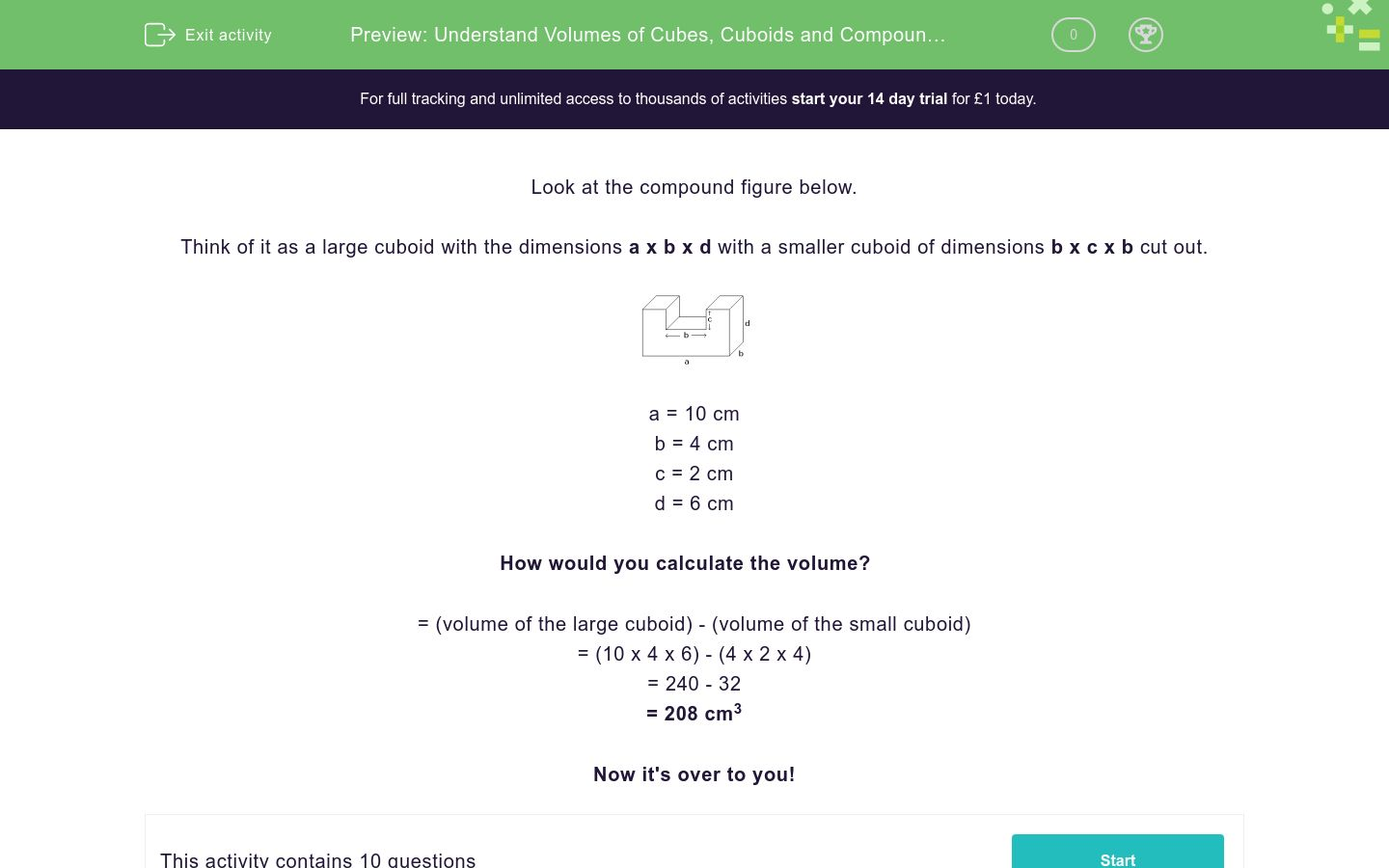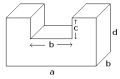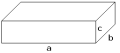# Volumes of Cubes, Cuboids and Compound Figures

In this worksheet, students find the volume of cubes, cuboids and compound figures.Key stage:  KS 4

Curriculum topic:  Geometry and Measures

Difficulty level:### QUESTION 1 of 10

 Look at this compound figure. Think of it as a large cuboid with dimensions a x b x d with a smaller cuboid of dimensions b x c x b cut out.a = 10 cm

b = 4 cm

c = 2 cm

d = 6 cm

Volume   = vol. of large cuboid - vol. of small cuboid.

= 10 x 4 x 6 - 4 x 2 x 4

= 240 - 32

= 208 cm3

 Find the volume of the following cuboid.a = 13 cm

b = 11 cm

c = 6 cm

Volume = ____ cm3

 Find the volume of the following cuboid.a = 11 cm

b = 9 cm

c = 5 cm

Volume = ____ cm3

 Find the volume of the following cuboid.a = 14 cm

b = 8 cm

c = 6 cm

Volume = ____ cm3

 Find the volume of the following compound figure.a = 10 cm

b = 12 cm

c = 6 cm

d = 10 cm

Volume = ____ cm3

 Find the volume of the following cube.c = 7 cm

Volume = ____ cm3

 Find the volume of the following compound figure,which is made of cuboids.a = 17 cm

b =12 cm

c = 4 cm

d =12 cm

Volume = ____ cm3

 Find the volume of the following compound figure,which is made of cuboids.a = 18 cm

b =12 cm

c = 6 cm

d = 7 cm

Volume = ____ cm3

 Find the volume of the following compound figure,which is made of cuboids.a = 14 cm

b =11 cm

c = 7 cm

d = 8 cm

Volume = ____ cm3

 Find the volume of the following cuboid.a = 11.5 cm

b = 9 cm

c = 8 cm

Volume = ____ cm3

 Find the volume of the following compound figure.a = 10 cm

b = 12.5 cm

c = 6.5 cm

d = 10 cm

Volume = ____ cm3

• Question 1
 Find the volume of the following cuboid.a = 13 cm

b = 11 cm

c = 6 cm

Volume = ____ cm3

858
• Question 2
 Find the volume of the following cuboid.a = 11 cm

b = 9 cm

c = 5 cm

Volume = ____ cm3

495
• Question 3
 Find the volume of the following cuboid.a = 14 cm

b = 8 cm

c = 6 cm

Volume = ____ cm3

672
• Question 4
 Find the volume of the following compound figure.a = 10 cm

b = 12 cm

c = 6 cm

d = 10 cm

Volume = ____ cm3

336
EDDIE SAYS
a x b x d - b x c x b
• Question 5
 Find the volume of the following cube.c = 7 cm

Volume = ____ cm3

343
• Question 6
 Find the volume of the following compound figure,which is made of cuboids.a = 17 cm

b =12 cm

c = 4 cm

d =12 cm

Volume = ____ cm3

1872
EDDIE SAYS
a x b x d - b x c x b
• Question 7
 Find the volume of the following compound figure,which is made of cuboids.a = 18 cm

b =12 cm

c = 6 cm

d = 7 cm

Volume = ____ cm3

864
EDDIE SAYS
a x b x d - a x c x c
• Question 8
 Find the volume of the following compound figure,which is made of cuboids.a = 14 cm

b =11 cm

c = 7 cm

d = 8 cm

Volume = ____ cm3

546
EDDIE SAYS
a x b x d - a x c x c
• Question 9
 Find the volume of the following cuboid.a = 11.5 cm

b = 9 cm

c = 8 cm

Volume = ____ cm3

828
• Question 10
 Find the volume of the following compound figure.a = 10 cm

b = 12.5 cm

c = 6.5 cm

d = 10 cm

Volume = ____ cm3

234.375
EDDIE SAYS
a x b x d - b x c x b
---- OR ----

Sign up for a £1 trial so you can track and measure your child's progress on this activity.

### What is EdPlace?

We're your National Curriculum aligned online education content provider helping each child succeed in English, maths and science from year 1 to GCSE. With an EdPlace account you’ll be able to track and measure progress, helping each child achieve their best. We build confidence and attainment by personalising each child’s learning at a level that suits them.

Start your £1 trial